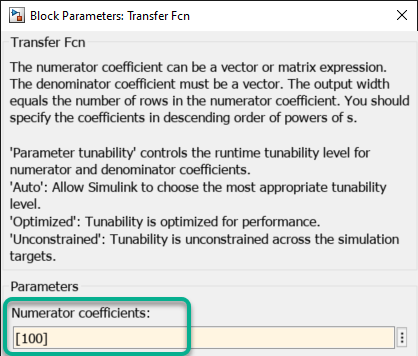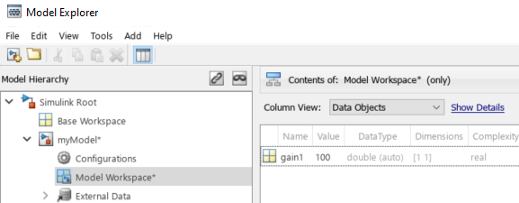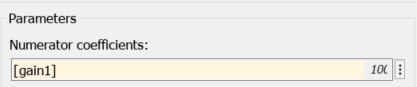## Specify Parameters for Estimation

### Choosing Which Parameters to Estimate First

Simulink® Design Optimization™ software lets you estimate scalar, vector, and matrix parameters. You can take an iterative approach to estimating model parameters. For example, if you have a large number of parameters to estimate, start by estimating those that most influence the output. After you estimate a subset of parameters and validate the estimated parameters, you can select the remaining parameters for estimation.

You can also first use sensitivity analysis to identify the parameters that most influence the estimation, and then specify these parameters for estimation. To open the Sensitivity Analyzer, in the Parameter Estimation tab, clickSensitivity Analysis. In the Sensitivity Analyzer, you can identify the model parameters that most influence the estimation problem and compute initial values for the estimation parameters.

### Add Model Parameters as Variables for Estimation

The software can only estimate variables that are in use by the model. Create variables for estimation in the MATLAB® or model workspace, and specify your Simulink model or block parameters using these variables.

In this figure, the Numerator coefficients parameter of a Transfer Fcn block is specified as a numerical value.To estimate the Numerator coefficients parameter, specify it as variable `gain1`:

1. Create the variable `gain1` in one of the following ways:

• Add the variables to the model workspace, and specify initial values.• Write initialization code in the PreloadFcn callback of the model. For more information, see Model Callbacks.

`gain1 = 100`

2. Specify the block parameter as variable `gain1` in the Transfer Fcn block dialog box.You can now select `gain1` for estimation. See, Specify Parameters for Estimation.

### Specify Parameters for Estimation

You can specify the parameters for estimation experiments using the Estimated Parameters editor. In the Parameter Estimator, on the Parameter Estimation tab, click Select Parameters.To select parameters for all experiments, click in the Parameters Tuned for all Experiments panel. This opens the Select model variables dialog. Here you can select the parameters you want to estimate by clicking the check box next to it or specifying an expression. For more information see Select Parameters Using Select Model Variables Dialog Box.

The editor looks likeFor example, in the `engine_idle_speed` model, select `freq1`, `freq2`, `freq3`, `gain1`, `gain2`, `gain3` and `mean_speed` for estimation. You do not need to estimate the parameters all at once. You can first select all the parameters you are interested in, and then later select a subset to estimate. By default, all the parameters are selected for estimation. To deselect the ones you do not want to estimate, clear the Estimate check box for a parameter. For this example, only estimate `gain1`, `gain2`, `gain3` and `mean_speed`. Set their initial values 10, 100, 50, and 500, respectively, and then click . The Edit: Estimated Parameters dialog box looks likeTo learn how to specify initial values and upper and lower bounds of the parameters, see Specifying Initial Guesses and Upper/Lower Bounds.

#### Select Parameters to Estimate for a Specific Experiment

To select the parameters to estimate in a specific experiment, first, select the experiment for estimation as described in Estimate Parameters and States. Then, you can use the Edit:Estimated Parameters dialog to select parameters to estimate for that experiment. Select the experiment name from the Experiment: combo box in the Parameters and Initial States Tuned per Experiment panel. Then click to launch the experiment editor for the experiment you select.

Alternatively, you can right click the experiment name in the Experiments list and select Edit.... In the experiment editor, click the button in the Parameters panel. In the Select model variables dialog box, you can select the parameters you want to estimate in this experiment by checking the box next to it or specifying an expression. For more information see Select Parameters Using Select Model Variables Dialog Box.

#### Select Parameters Using Select Model Variables Dialog Box

Use this dialog box to specify parameters to estimate. The table lists the variables that the model uses to set block parameter values. The variables can reside in the model workspace, the base workspace, or a data dictionary.

Select variables by clicking the check box next to each variable. If your model contains many variables, filter the list by typing in the Filter by variable name field. The Used By column lists all blocks in the model that use the variable. When a variable is used in more than one block, all blocks are listed. To highlight blocks in the model that use the variable, click the block name.The variables that you select must have a numeric value that uses the data type `double`. If the value of a variable is not a `double` number, use these techniques:

• To select a single element or a subset of a matrix or array variable, click Specify expression indexing if necessary.Enter an expression such as `myArray(2)`, which selects the second element of an array variable `myArray`.

After you type the expression, press the Enter key to add the variable to the list of model variables.

• To use a variable of a numeric data type other than `double`, convert the variable to a `Simulink.Parameter` object, which separates a parameter value from its data type. Set the `Value` property to a default `double` number, and use the `DataType` property to control the data type.

• To use the value of a `Simulink.Parameter` object, specify the `Value` property. Enter the expression `myParamObj.Value`.

• To use a numeric field of a structure, enter `myStruct.PID.P1`. If you store the structure in a `Simulink.Parameter` object, enter `myStruct.Value.PID.P1`.

• To use one cell of a cell array, enter `myCells{3}`.

You cannot use mathematical expressions such as a + b. Sometimes, models have parameters that are not explicitly defined in the model itself. For example, a gain `k` could be defined in the MATLAB workspace as `k = a + b`, where `a` and `b` are not defined in the model but `k` is used. To add these independent parameters, see Add Model Parameters as Variables for Estimation.

#### Specifying Initial Guesses and Upper/Lower Bounds

After you select parameters, you can specify

• Initial guess — The value the estimation uses to start the process.

• Minimum — The smallest allowable parameter value. The default is `-Inf`.

• Maximum — The largest allowable parameter value. The default is `+Inf`.

You can enter the initial value in the dialog box below the parameter name. You can specify the minimum and maximum value fields by clicking the arrow . The default minimum and maximum values are `-Inf` and `+Inf`, respectively, but you can select any range you want.If you believe a parameter lies within a finite range, it is best not to use the default minimum and maximum values. Often, there are computational advantages in specifying finite bounds. It can be very important to specify lower and upper bounds. For example, if a parameter specifies the weight of a part, be sure to specify `0` as the absolute lower bound if better information is unavailable.

Note

When you specify the minimum and maximum values for the parameters, it does not affect your settings in the Parameters list under Data Browser pane. You make these choices for each experiment.

• Scale — The scale value to use for normalization. The parameters are scaled, or normalized, by dividing their current value by the scale value. Scale is useful, in situations, for example, when parameters have different orders of magnitude.

The default scale value is the next power of 2 greater than the current value of the parameter. For example, if the current parameter value is `15`, Scale is `16` ( =${2}^{4}$). You can edit this field to provide an alternate scaling factor.

## Related Examples